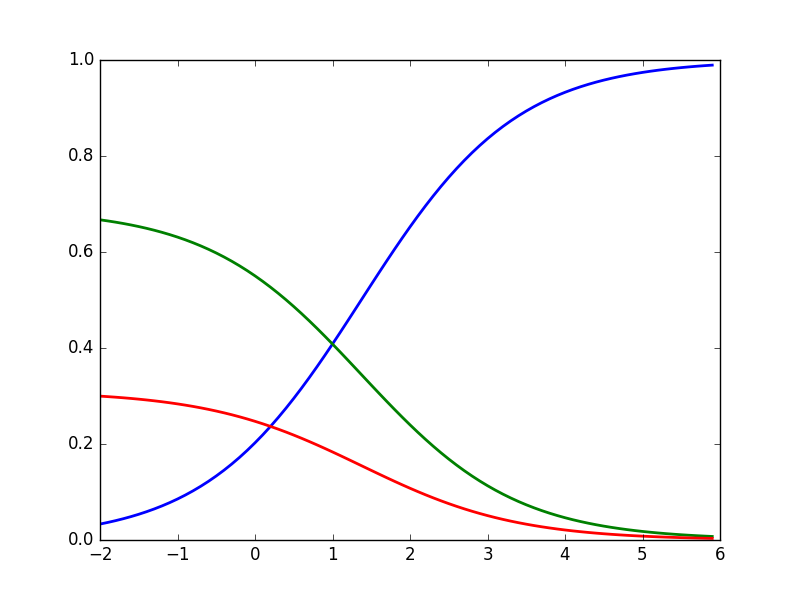# Softmax function

Alex Egg,

In machine learning, a softmax function turns scores (the output of a logistic regression classifier for example) into probabilities.

It can take any type of scores and turn them into proper probabilities, meaning that they sum up to 1.

In the python example below let’s presume we have a classifier which out puts 3 scores for 3 classes. Let’s see what probabilities the softmax function gives us:

"""Softmax.py"""

scores = [3.0, 1.0, 0.2]

import numpy as np

def softmax(x):
"""Compute softmax values for each sets of scores in x."""
return np.exp(x) / np.sum(np.exp(x), axis=0)

print(softmax(scores))

# Plot softmax curves
import matplotlib.pyplot as plt
x = np.arange(-2.0, 6.0, 0.1)
scores = np.vstack([x, np.ones_like(x), 0.2 * np.ones_like(x)])

plt.plot(x, softmax(scores).T, linewidth=2)
plt.show()

[ 0.8360188   0.11314284  0.05083836]You can see as per out assumption, the probabilities of the 3 classes sum up to 1.